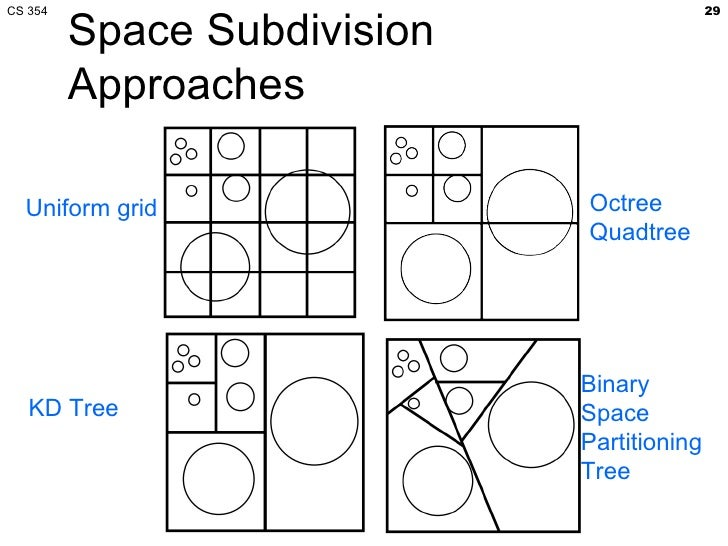# Is there any way of ordering/sorting vectors?

I am working on KD-Tree for nearest neighbor algorithm, where at each level of the tree we arbitrarily choose a dimension to cut upon and sort the points based on that chosen dimension value, after which we can choose mid-point to split upon such that dividing the points from that middle point will give us a balanced tree (has same number of points in either of the subtrees). At each level a single dimension is chosen and process is repeated.

For example: for data having dimensions (x,y,z) let's say first level of the tree 'X' is chosen at cut dimension, 'Y' at second level, 'Z' at third and then repeated again with 'X' again at fourth level and so on. So for first level all points are sorted according to dimension 'X' and split into left and right subtree based on the middle value of the sorted data.

I am sure you got the gist of it. Otherwise please read more here. KD-Tree (Wiki)

Now what I want to try something where rather than using each dimension at different level, I use all at the same level. i.e. I take points p(x,y,z) as vectors. But as you can guess if I do this, I have no way of ordering (not any I know of) the vectors (points) such that I can find a vector to divide upon hence still keeping my tree somewhat balanced.

PS: I am not much concerned about if it is actually a better or worse way of doing thing. Right now it's more about if it is possible to do it this way and then find out why it is better or worse.

Edit: Just to make it clear. Basically I want to be able to have an ordering which won't destroy the order based on distances. i.e. if a point/vector A has less distance from another point P, it comes first in the order than some farther point, let's say B. My end goal is to use this to try and create some kind of nearest neighbor algorithm.

• It would help you to get answers if you added which environment you are working in (R? Python? etc.). Nov 18, 2018 at 3:27
• @RichardCareaga I am currently exploring python, though my question is mostly research based. So that if there is a way to order vectors I would like to know it to be able to use it for my purpose. But if there is an environment specific solution too, I would be happy to know. Thanks for the comment. Nov 18, 2018 at 9:23

If I understand your question correctly, you're looking for something that another (beyond KD-trees) standard space partitioning algorithm does. This one is called BSP-trees (for Binary Space Partition) and it uses vectors instead of dimensions for its space subdivision/partitioning. You can see it contrasted to KD-trees in slide 29 (previewed below) and beyond of this presentation.The criterion that is used in the algorithm is intended to balance the data in the branches of the tree. This guides the definition of an order on vectors for what seems to be your purpose as well.

Otherwise, more generally speaking, one way to define a total order on a vector space is by identifying an order vector. Then your ordering is defined by the dot product with this vector. This represents projecting the entire space on the line defined by the vector and thus using the total order of the one-dimensional space it defines. For a more detailed explanation of the dot product, consider this video.

• Appreciate the help. I will read on the information provided by you and see if it helps me for what I am looking for. I will let you know :) Dec 26, 2018 at 12:06
• can you explain a bit about what you mean by "identifying an order vector", or guide me somewhere I can read about how it can be done. Are you suggesting for an arbitrary ordering vector to be chosen and sort every vector according to the dot product of that vector and order vector? Thanks in advance. Dec 28, 2018 at 7:22
• Yes, but I wouldn't call it "arbitrary". You actually choose it in order to determine the direction of the order Dec 31, 2018 at 13:57
• @AshokSuthar, I've added a link to a video that visually explains how the dot product serves as a total order on a vector space. Jan 2, 2019 at 8:35
• Sorry for being so late. I understand how it can work, but am unable to understand how I can find an order vector? i.e. let's say I have some data, in terms of vectors. How do I find an order vector which can sort that accordingly? Is there something I am missing? Feb 14, 2019 at 11:40